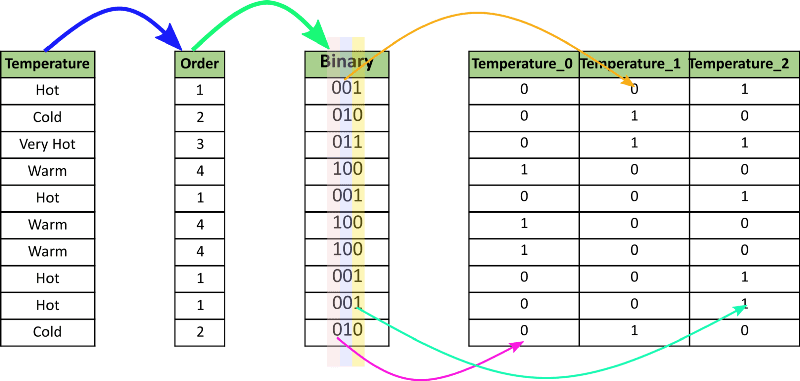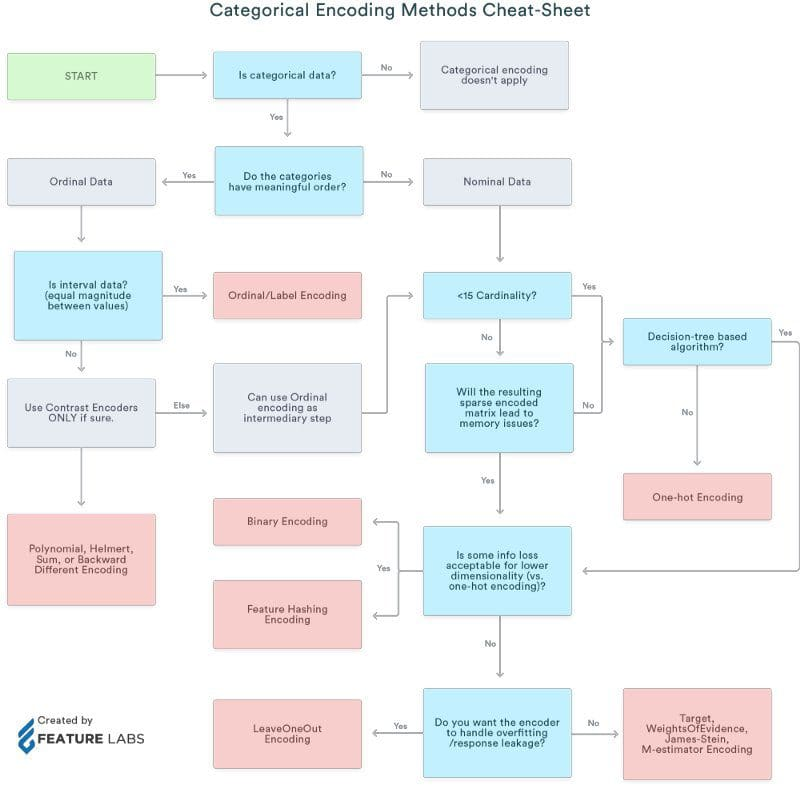# How to Deal with Categorical Data for Machine Learning

Check out this guide to implementing different types of encoding for categorical data, including a cheat sheet on when to use what type.In this blog we will explore and implement:

• One-hot Encoding using:
• Python’s category_encoding library
• Scikit-learn preprocessing
• Pandas' get_dummies
• Binary Encoding
• Frequency Encoding
• Label Encoding
• Ordinal Encoding

# What is Categorical Data?

Categorical data is a type of data that is used to group information with similar characteristics, while numerical data is a type of data that expresses information in the form of numbers.

Example of categorical data: gender

Why do we need encoding?

• Most machine learning algorithms cannot handle categorical variables unless we convert them to numerical values
• Many algorithm’s performances even vary based upon how the categorical variables are encoded

Categorical variables can be divided into two categories:

• Nominal: no particular order
• Ordinal: there is some order between values

We will also refer to a cheat sheet that shows when to use which type of encoding.

# Method 1: Using Python’s Category Encoder Library

category_encoders is an amazing Python library that provides 15 different encoding schemes.

Here is the list of the 15 types of encoding the library supports:

• One-hot Encoding
• Label Encoding
• Ordinal Encoding
• Helmert Encoding
• Binary Encoding
• Frequency Encoding
• Mean Encoding
• Weight of Evidence Encoding
• Probability Ratio Encoding
• Hashing Encoding
• Backward Difference Encoding
• Leave One Out Encoding
• James-Stein Encoding
• M-estimator Encoding
• Thermometer Encoder

Importing libraries:

```import pandas as pd
import sklearn

pip install category_encoders

import category_encoders as ce```

Creating a dataframe:

```data = pd.DataFrame({ 'gender' : ['Male', 'Female', 'Male', 'Female', 'Female'],
'class' : ['A','B','C','D','A'],
'city' : ['Delhi','Gurugram','Delhi','Delhi','Gurugram'] })Image By Author

Implementing one-hot encoding through category_encoder

In this method, each category is mapped to a vector that contains 1 and 0 denoting the presence or absence of the feature. The number of vectors depends on the number of categories for features.

Create an object of the one-hot encoder:

```ce_OHE = ce.OneHotEncoder(cols=['gender','city'])

data1 = ce_OHE.fit_transform(data)Image By Author

Binary Encoding

Binary encoding converts a category into binary digits. Each binary digit creates one feature column.Image Ref

```ce_be = ce.BinaryEncoder(cols=['class']);

# transform the data
data_binary = ce_be.fit_transform(data["class"]);
data_binary```Image By Author

Similarly, there are another 14 types of encoding provided by this library.

# Method 2: Using Pandas' Get Dummies

`pd.get_dummies(data,columns=["gender","city"])`Image By Author

We can assign a prefix if we want to, if we do not want the encoding to use the default.

`pd.get_dummies(data,prefix=["gen","city"],columns=["gender","city"])`Image By Author

# Method 3: Using Scikit-learn

Scikit-learn also has 15 different types of built-in encoders, which can be accessed from sklearn.preprocessing.

## Scikit-learn One-hot Encoding

Let's first get the list of categorical variables from our data:

```s = (data.dtypes == 'object')
cols = list(s[s].index)

from sklearn.preprocessing import OneHotEncoder

ohe = OneHotEncoder(handle_unknown='ignore',sparse=False)```

Applying on the gender column:

```data_gender = pd.DataFrame(ohe.fit_transform(data[["gender"]]))

data_gender```Image By Author

Applying on the city column:

```data_city = pd.DataFrame(ohe.fit_transform(data[["city"]]))

data_city```Image By Author

Applying on the class column:

```data_class = pd.DataFrame(ohe.fit_transform(data[["class"]]))

data_class```Image By Author

This is because the class column has 4 unique values.

Applying to the list of categorical variables:

```data_cols = pd.DataFrame(ohe.fit_transform(data[cols]))

data_cols```Image By Author

Here the first 2 columns represent gender, the next 4 columns represent class, and the remaining 2 represent city.

## Scikit-learn Label Encoding

In label encoding, each category is assigned a value from 1 through N where N is the number of categories for the feature. There is no relation or order between these assignments.

```from sklearn.preprocessing import LabelEncoder

le = LabelEncoder()
Label encoder takes no arguments
le_class = le.fit_transform(data[["class"]])```

Comparing with one-hot encoding

`data_class`Image By Author

## Ordinal Encoding

Ordinal encoding’s encoded variables retain the ordinal (ordered) nature of the variable. It looks similar to label encoding, the only difference being that label coding doesn't consider whether a variable is ordinal or not; it will then assign a sequence of integers.

Example: Ordinal encoding will assign values as Very Good(1) < Good(2) < Bad(3) < Worse(4)

First, we need to assign the original order of the variable through a dictionary.

```temp = {'temperature' :['very cold', 'cold', 'warm', 'hot', 'very hot']}
df=pd.DataFrame(temp,columns=["temperature"])
temp_dict = {'very cold': 1,'cold': 2,'warm': 3,'hot': 4,"very hot":5}
df```Image By Author

Then we can map each row for the variable as per the dictionary.

```df["temp_ordinal"] = df.temperature.map(temp_dict)
df```Image By Author

## Frequency Encoding

The category is assigned as per the frequency of values in its total lot.

`data_freq = pd.DataFrame({'class' : ['A','B','C','D','A',"B","E","E","D","C","C","C","E","A","A"]})`

Grouping by class column:

`fe = data_freq.groupby("class").size()`

Dividing by length:

`fe_ = fe/len(data_freq)`

Mapping and rounding off:

```data_freq["data_fe"] = data_freq["class"].map(fe_).round(2)
data_freq```Image By Author

In this article, we saw 5 types of encoding schemes. Similarly, there are 10 other types of encoding which we have not looked at:

• Helmert Encoding
• Mean Encoding
• Weight of Evidence Encoding
• Probability Ratio Encoding
• Hashing Encoding
• Backward Difference Encoding
• Leave One Out Encoding
• James-Stein Encoding
• M-estimator Encoding
• Thermometer Encoder

# Which Encoding Method is Best?

There is no single method that works best for every problem or dataset. I personally think that the get_dummies method has an advantage in its ability to be implemented very easily.

If you want to read about all 15 types of encoding, here is a very good article to refer to.

Here is a cheat sheet on when to use what type of encoding:Image Ref

## References:

Shelvi Garg is a Data Scientist at Spinny

By subscribing you accept KDnuggets Privacy Policy

By subscribing you accept KDnuggets Privacy Policy

By subscribing you accept KDnuggets Privacy Policy